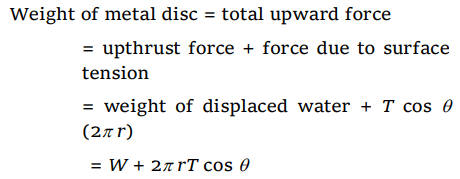## Mechanical Properties of Fluids Questions and Answers Part-4

1.When a drop of water is dropped on oil surface, then
a) It will mix up with oil
b) It spreads in the form of a film
c) It will deform
d) It remains spherical

Explanation: Because surface tension of water > surface tension of oil

2. Two pieces of glass plate one upon the other with a little water in between them cannot be separated easily because of
a) Inertia
b) Pressure
c) Surface tension
d) Viscosity

Explanation: Surface tension pulls the plates towards each other.

3. Small liquid drops assume spherical shape because
a) Atmospheric pressure exerts a force on a liquid drop
b) Volume of a spherical drop is minimum
c) Gravitational force acts upon the drop
d) Liquid tends to have the minimum surface area due to surface tension

Explanation: Sphere has the minimum surface area for the given volume of the liquid

4. A thin metal disc of radius r floats on water surface and bends the surface downwards along the perimeter making an angle $\theta$ with vertical edge of the disc. If the disc displaces a weight of water W and surface tension of water is T, then the weight of metal disc is
a) $2\pi r T+W$
b) $2\pi r T\cos\theta-W$
c) $2\pi r T\cos\theta+W$
d) $W-2\pi r T\cos\theta$

Explanation:5. A 10 cm long wire is placed horizontally on the surface of water and is gently pulled up with a force of $2\times 10^{-2} N$   to keep the wire in equilibrium. The surface tension, in Nm-1, of water is
a) 0.1
b) 0.2
c) 0.001
d) 0.002

Explanation:6. It is easy to wash clothes in hot water because its
a) Surface tension is more
b) Surface tension is less
c) Consumes less soap
d) None of these

Explanation: Surface tension of water decrease with rise in temperature

7. Due to which property of water, tiny particles of camphor dance on the surface of water
a) Viscosity
b) Surface tension
c) Weight
d) Floating force

Explanation: Surface tension

8. The force required to separate two glass plates of area $10^{-2} m^{2}$   with a film of water 0.05 mm thick between them, is (Surface tension of water is $70\times 10^{-3} N\diagup m$    )
a) 28 N
b) 14 N
c) 50 N
d) 38 N

Explanation: Force required to separate the plates9. Oil spreads over the surface of water whereas water does not spread over the surface of the oil, due to
a) Surface tension of water is very high
b) Surface tension of water is very low
c) Viscosity of oil is high
d) Viscosity of water is high